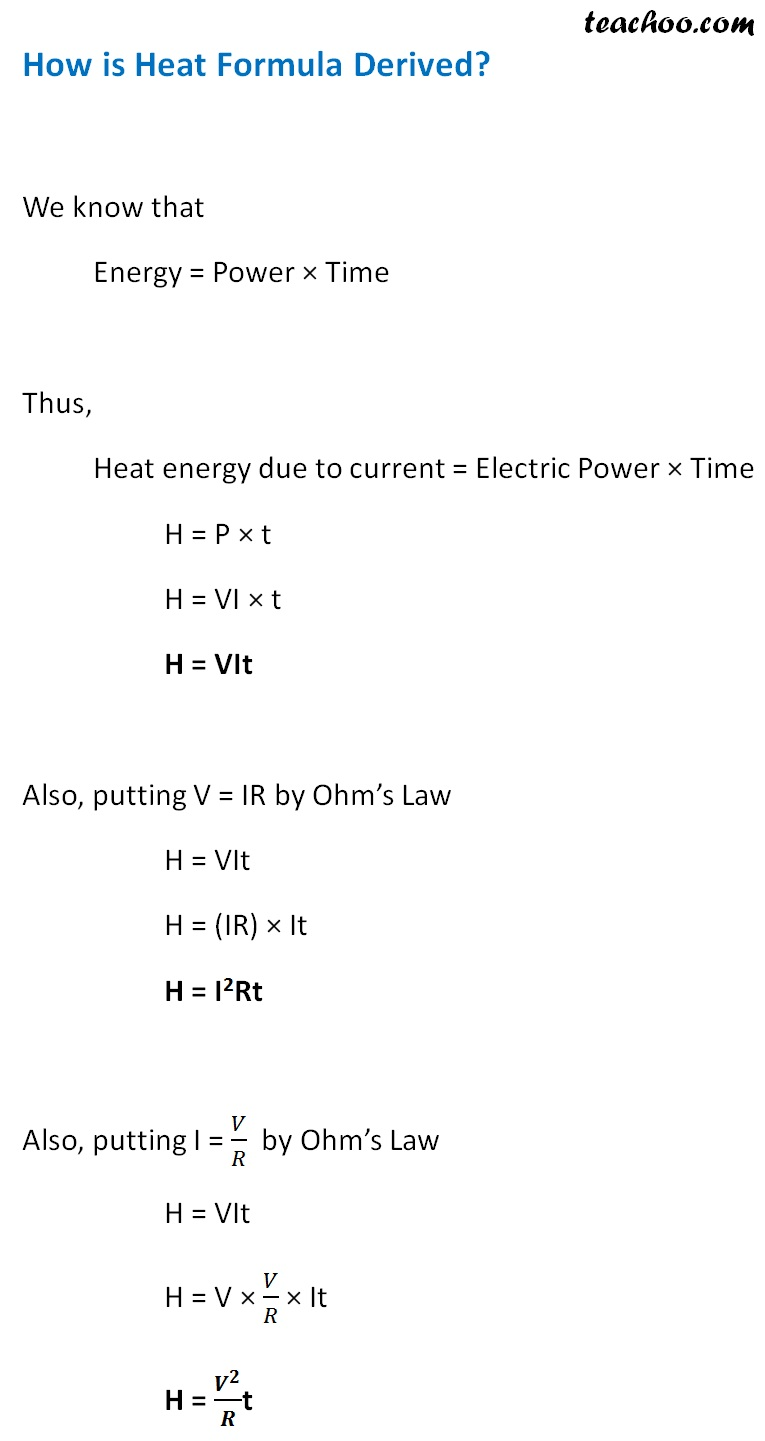Concepts

Class 10
Chapter 12 Class 10 - Electricity

## What is Electric Energy

Electric Energy is product of Electric Power and Time

Electric Energy = Electric power × Time

## What are Units of Electrical Energy?

It is Measured in Watt second or Joule

Explanation

Electric Energy = Electric power × Time

Electric Power is measured in Watt and Time in Second

So Electrical Energy-Watt × Hours = Watt Second

## Meaning of Heating Effect

When an electric current passes through a conductor (like a high resistance wire)

the conductor becomes hot after some time and produces heat

This is called heating effect of Electric Current

Example 1

A bulb becomes hot after its use for some time.This is because of heating effect of electric current

Example 2

When we switch on an electric iron,it becomes hot.This is also because of heating effect of electric current

## What causes Heating Effect of Electric Current

Heating Effect happens due to conversion of electric energy into heat energy

Explanation

We know that battery or cell is a source of electrical energy

Due to chemical reaction in this battery or cell,potential difference is generated

This potential difference causes electrons to flow through circuit

This circuit has resistors which resist flow of current

Work is to be done to overcome this resistance

While doing this work,

This source energy in conductor is dissipated(converted) in resistor as heat energy

H = I 2 RT

## How is Heat Formula DerivedThis is known as Joule's law of heating.

The law implies that

the heat produced in a resistor is

1. directly proportional to the square of current for a given resistance
It means if we double the current,the heat becomes 4 times
if we half the current,the heat becomes 1/4 times
Hence,more the current,more the heat. Less the current, less heat produced.

2. directly proportional to resistance for a given current
It means if we use a wire made of metal having more resistance (like nichrome wire),it will produce more heat
But if we use wire made of metal having less resistance (like copper),it will produce less heat

3. directly proportional to the time for which the current flows through the resistor
It means if we switch on an electric gadget for more time,it will get heated up more
But if we use an electric gadget for less time (switch it off after use),it will get less heated

## Why do some electric appliances get heated up more (like electric iron) while others get heated up less(like TV)

It is because electric iron has a heating element like high resistance wire which help in converting most of electric energy into heat energy

Explanation

We know that as per Joule's Law of Heating

Heat is Directly Proportional to the resistance of the wire for a given current

It means if we use a wire made of metal having more resistance (like nichrome wire),it will resist the flow of current and hence it will produce more heat.

Since the resistance of the wires used in electric iron is more than that used Tv, more heat is produced in an electric iron.

Such kind of nichrome wire is used in electric iron which acts as heating element

This converts most of electric energy into heat energy

## Different formulas for Heat

We know that,

Heat = Electrical power x time

Hence, the three formulae become

1. H = (VI)t
2. H = (I 2 R)t
3. H = (V 2 /R)t

## Questions

Example 12.11 - 100 J of heat is produced each second in a 4 Ω resistance. Find the potential difference across the resistor.

Question 8 - In an electrical circuit two resistors of 2 Ω and 4 Ω respectively
are connected in series to a 6 V battery. The heat dissipated by
the 4 Ω resistor in 5 s will be

(a) 5 J
(b) 10 J
(c) 20 J
(d) 30 J

In an electrical circuit two resistors of 2 Ω and 4 Ω respectively
are connected in parallel to a 6 V battery. The heat dissipated by
the 4 Ω resistor in 5 s will be
(a) 45 J
(b) 20 J
(c) 60 J
(d) 35 J

Learn in your speed, with individual attention - Teachoo Maths 1-on-1 Class

### Transcript

How is Heat Formula Derived? We know that Energy = Power × Time Thus, Heat energy due to current = Electric Power × Time H = P × t H = VI × t H = VIt Also, putting V = IR by Ohm’s Law H = VIt H = (IR) × It H = I2Rt Also, putting I = 𝑉/𝑅 by Ohm’s Law H = VIt H = V × 𝑉/𝑅 × It H = 𝑽^𝟐/𝑹t